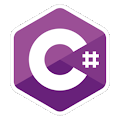Programming Course in C# ¡Free!

# Use of {0} and comments

Proposed exercise

Write a C# program to ask the user for three numbers and display their multiplication. The first line must be a comment with your name and surname. It MUST look as follows:

Enter the first number to multiply
12
Enter the second number to multiply
23
Enter the third number to multiply
2

Result: 12 x 23 x 2 = 552

Output

Solution

```using System;
public class Multiplication
{
public static void Main()
{
int number1, number2, number3;

Console.Write("Enter the first number to multiply: ");